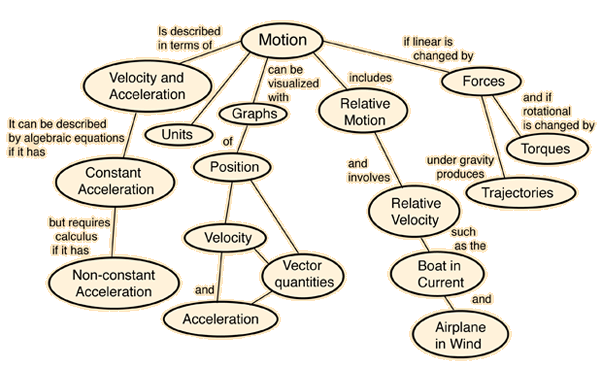Index

Newton's Laws

 HyperPhysics***** Mechanics R Nave
Go Back

# Description of Motion in One Dimension

Motion is described in terms of displacement (x), time (t), velocity (v), and acceleration (a). Velocity is the rate of change of displacement and the acceleration is the rate of change of velocity. The average velocity and average acceleration are defined by the relationships: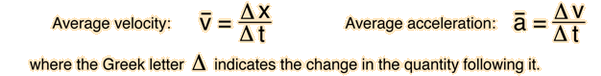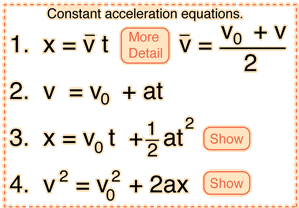A bar above any quantity indicates that it is the average value of that quantity. If the acceleration is constant, then equations 1,2 and 3 represent a complete description of the motion. Equation 4 is obtained by a combination of the others. Click on any of the equations for an example.

 Graphing one-dimensional motion
Index

Motion concepts

 HyperPhysics***** Mechanics R Nave
Go Back

# Distance, Average Velocity and Time

The case of motion in one dimension (one direction) is a good starting point for the description of motion. Perhaps the most intuitive relationship is that average velocity is equal to distance divided by time:Index

Motion concepts

Motion example

 HyperPhysics***** Mechanics R Nave
Go Back

# Distance, Average Velocity and Time

The case of motion in one dimension (one direction) is a good starting point for the description of motion. A basic type of calculation may be explored here by substituting numbers and then clicking on the bold text of the quantity you wish to calculate. Make only one substitution at a time and click the desired quantity -- then you can repeat with other substitutions.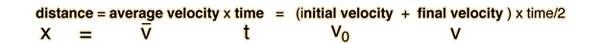m = m/s * s = ( m/s + m/s) * time/2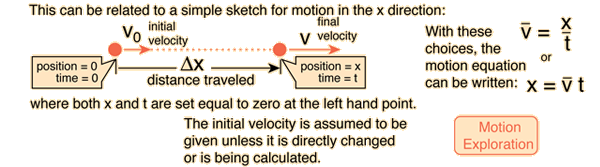Index

Motion concepts

 HyperPhysics***** Mechanics R Nave
Go Back

# Forms of Motion Equations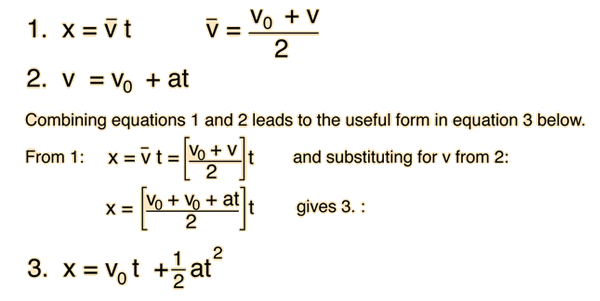Alternate derivation using calculus
Index

Motion concepts

Motion example

 HyperPhysics***** Mechanics R Nave
Go Back

# Forms of Motion EquationsIndex

Motion concepts

 HyperPhysics***** Mechanics R Nave
Go Back

# Motion Example

Initial velocity =m/s, Final velocity =m/sDistance traveled x = m
 In this example, the items labeled on the diagram are considered primary: if one of them is changed, the others remain the same. The data in the boxes may be changed, and the calculation will be done when you click outside the box, subject to the constraints described. Changing average velocity, acceleration or time will force a change in at least one of the original quantities. In this version, the final velocity is allowed to change. Distance x = m Initial velocity v0 = m/s Final velocity v = m/s Average velocity = m/s Acceleration a = m/s^2 Time t = sIndex

Motion concepts

 HyperPhysics***** Mechanics R Nave
Go Back

# Motion Example

Initial velocity =m/s, Final velocity =m/sDistance traveled x = m
 In this example, the items labeled on the diagram are considered primary: if one of them is changed, the others remain the same. The data in the boxes may be changed, and the calculation will be done when you click outside the box, subject to the constraints described. If the average velocity is directly changed, the final velocity is adjusted for consistency. If the acceleration or time is changed, then the distance is allowed to change. Distance x = m Initial velocity v0 = m/s Final velocity v = m/s Average velocity = m/s Acceleration a = m/s^2 Time t = s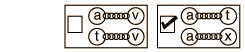Index

Motion concepts

 HyperPhysics***** Mechanics R Nave
Go Back

# Linear Motion Explorer

These motion equations apply only in the case of constant acceleration. It is assumed that x=0 at t=0 and that the motion is being examined at time t. After you have edited any box of motion data, click on the text or symbol for the quantity you wish to calculate. If it does not behave the way you expect, see the comments on the calculation.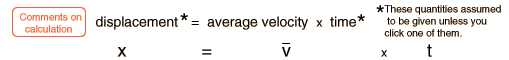m     =         m/s         x s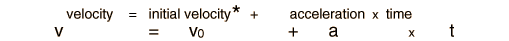m/s = m/s +         m/s2 x s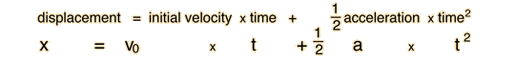m = m/s x s + 1/2 m/s2 * t2
Index

Motion concepts

 HyperPhysics***** Mechanics R Nave
Go Back